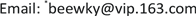﻿ 延迟随机游动的超出的局部渐近性质 The Local Asymptotics of the Overshoot of a Delay Random Walk

Statistics and Application
Vol. 08  No. 02 ( 2019 ), Article ID: 29914 , 5 pages
10.12677/SA.2019.82041

The Local Asymptotics of the Overshoot of a Delay Random Walk

Tiantian Hu, Zhengmin Ji, Yanzhu Mao, Kaiyong Wang*

School of Mathematics and Physics, Suzhou University of Science and Technology, Suzhou JiangsuReceived: Apr. 4th, 2019; accepted: Apr. 19th, 2019; published: Apr. 26th, 2019ABSTRACT

This paper investigates a delay random walk. Using the renewal equation and the results of random walk with zero delay, when the distribution of the increment of the random walk has a heavy tail, the paper obtains the local asymptotics of the overshoot of the delay random walk.

Keywords:Random Walk, Delay, Overshoot, Local AsymptoticsCopyright © 2019 by authors and Hans Publishers Inc.

This work is licensed under the Creative Commons Attribution International License (CC BY).

http://creativecommons.org/licenses/by/4.0/1. 引言

$\left\{{X}_{i},i\ge 2\right\}$ 为独立同分布 $\left(i.i.d\right)$ 的随机变量具有共同的分布 $K$ 及有限的负均值 ${\mu }_{k}=-m$ 。随机变量 ${X}_{1}$$\left\{{X}_{i},i\ge 2\right\}$ 独立且具有分布 ${K}_{1}$ 。由 $\left\{{X}_{i},i\ge 1\right\}$ 定义了随机游动 $\left\{{S}_{n},n\ge 0\right\}$ ，其中，设 ${S}_{0}=0$${S}_{n}=\underset{i=1}{\overset{n}{\sum }}{X}_{i}$$n\ge 1$ ，称 $\left\{{S}_{n},n\ge 0\right\}$ 为由 $\left\{{X}_{i},i\ge 1\right\}$ 产生的随机游动。当 ${X}_{1}$$\left\{{X}_{i},i\ge 2\right\}$ 具有不同分布时，称此随机游动为延迟的随机游动，否则称为零延迟的随机游动。记

$\eta \left(x\right)=\mathrm{inf}\left\{n\ge 1:{S}_{n}>x\right\}$$x\ge 0$

${Z}_{+}=\eta \left(0\right)$${S}_{{Z}_{+}}$ 为首次上穿梯高。周知，当 $0 时， ${Z}_{+}$${S}_{{Z}_{+}}$ 为亏损随机变量，即

$P\left({S}_{{Z}_{+}}<\infty \right)=P\left({Z}_{+}<\infty \right)=q\in \left(0,1\right)$

${K}_{+}$${S}_{{Z}_{+}}$ 的分布，记

${K}_{{P}_{+}}\left(x\right)=P\left({S}_{{Z}_{+}}\le x|{Z}_{+}<\infty \right)=\frac{{K}_{+}\left(x\right)}{q},x\ge 0$

$\stackrel{¯}{{K}_{{P}_{+}}}\left(x\right)=1-\frac{{K}_{+}\left(x\right)}{q}={q}^{-1}\left(q-{K}_{+}\left(x\right)\right)={q}^{-1}\stackrel{¯}{{K}_{+}}\left(x\right),x\ge 0$

$\stackrel{¯}{V}\left(x+y\right)~\stackrel{¯}{V}\left(x\right)$

$\stackrel{¯}{{V}^{*2}}\left(x\right)~2\stackrel{¯}{V}\left(x\right)$

${\int }_{0}^{x}\stackrel{¯}{V}\left(x-y\right)\stackrel{¯}{V}\left(y\right)\text{d}y~2\stackrel{¯}{V}\left(x\right){\int }_{0}^{x}\stackrel{¯}{V}\left(y\right)\text{d}y$

$V$ 是支撑在 $\left(-\infty ,\infty \right)$ 上的分布，称 $V$ 属于某一分布族，若 $V\left(x\right){Ι}_{\left\{x>0\right\}}$ 属于某一分布族，其中 ${Ι}_{A}$$A$ 的示性函数。

${S}^{*}\subset S\subset L$

$P\left({S}_{\eta \left(x\right)}\in x+{\Delta }_{T}\right)~{\left(\left(1-q\right)}^{-1}{\int }_{0}^{T}\stackrel{¯}{{K}_{+}}\left(y\right)\text{d}y+T\right){m}^{-1}\stackrel{¯}{K}\left(x\right)$ (1.1)

2. 主要结果的证明

${H}_{V}=\left\{h:\left[0,\infty \right)\to \left[0,\infty \right):h\left(x\right)↑\infty ,{x}^{-1}h\left(x\right)\to 0且\stackrel{¯}{V}\left(x-y\right)~\stackrel{¯}{V}\left(x\right)对|y|\le h\left(x\right)一致\right\}.$

$V\in L⇔{H}_{V}\ne \varphi$

$P\left({S}_{\eta \left(x\right)}\in x+{\Delta }_{T}\right)~{\left(\left(1-q\right)}^{-1}{\int }_{0}^{T}\stackrel{¯}{{K}_{+}}\left(y\right)\text{d}y+T\right){m}^{-1}\stackrel{¯}{K}\left(x\right)$

${{S}^{\prime }}_{n}={S}_{n}-{X}_{1}$$n\ge 1$

${\eta }^{\prime }\left(x\right)=\mathrm{inf}\left\{n\ge 1:{{S}^{\prime }}_{n}>x\right\}$$x\ge 0$

$\begin{array}{l}P\left({S}_{\eta \left(x\right)}\in x+{\Delta }_{T}\right)\\ ={K}_{1}\left(x+{\Delta }_{T}\right)+\left({\int }_{-\infty }^{h\left(x\right)}+{\int }_{h\left(x\right)}^{x-h\left(x\right)}+{\int }_{x-h\left(x\right)}^{x}\right)P\left({{S}^{\prime }}_{{\eta }^{\prime }\left(x-y\right)}\in x-y+{\Delta }_{T}\right){K}_{1}\left(\text{d}y\right)\\ =:{K}_{1}\left(x+{\Delta }_{T}\right)+\underset{i=1}{\overset{3}{\sum }}{J}_{i}\left(x\right)\end{array}$ (2.1)

${K}_{1}\left(x+{\Delta }_{T}\right)=o\left(1\right)\stackrel{¯}{{K}_{1}}\left(x\right)=o\left(1\right)\stackrel{¯}{K}\left(x\right)$ (2.2)

$P\left({{S}^{\prime }}_{{\eta }^{\prime }\left(x\right)}\in x+{\Delta }_{T}\right)~{C}_{1}\stackrel{¯}{K}\left(x\right)$ (2.3)

$\begin{array}{c}{J}_{1}\left(x\right)~{C}_{1}{\int }_{-\infty }^{h\left(x\right)}\stackrel{¯}{K}\left(x-y\right){K}_{1}\left(\text{d}y\right)\\ \text{~}{C}_{1}\stackrel{¯}{K}\left(x\right).\end{array}$ (2.4)

$\begin{array}{c}{J}_{2}\left(x\right)~{C}_{1}{\int }_{h\left(x\right)}^{x-h\left(x\right)}\stackrel{¯}{K}\left(x-y\right){K}_{1}\left(\text{d}y\right)\\ \le {C}_{1}\left(\stackrel{¯}{K}\left(x-h\left(x\right)\right)\stackrel{¯}{{K}_{1}}\left(h\left(x\right)\right)+{\int }_{h\left(x\right)}^{x-h\left(x\right)}\stackrel{¯}{{K}_{1}}\left(x-y\right)K\left(\text{d}y\right)\right)\\ =O\left(1\right)\left(\stackrel{¯}{K}\left(x-h\left(x\right)\right)\stackrel{¯}{{K}_{1}}\left(h\left(x\right)\right)+{\int }_{h\left(x\right)}^{x-h\left(x\right)}\stackrel{¯}{K}\left(x-y\right)K\left(\text{d}y\right)\right)\\ =o\left(1\right)\stackrel{¯}{K}\left(x\right)\end{array}$ (2.5)

$\begin{array}{c}{J}_{3}\left(x\right)\le \stackrel{¯}{{K}_{1}}\left(x-h\left(x\right)\right)-\stackrel{¯}{{K}_{1}}\left(x\right)\\ =o\left(1\right)\stackrel{¯}{{K}_{1}}\left(x\right)=o\left(1\right)\stackrel{¯}{K}\left(x\right)\end{array}$ (2.6)

The Local Asymptotics of the Overshoot of a Delay Random Walk[J]. 统计学与应用, 2019, 08(02): 370-374. https://doi.org/10.12677/SA.2019.82041

1. 1. Janson, S. (1986) Moments for First-Passage and Last-Exit Times, the Minimum, and Related Quantities for Random Walks with Positive Drift. Advances in Applied Probability, 18, 865-879. https://doi.org/10.2307/1427253

2. 2. Borovkov, A.A. and Foss, S. (2000) Estimates for Overshooting an Arbi-trary Boundary by a Random Walk and Their Applications. Theory of Probability & Its Applications, 44, 231-253. https://doi.org/10.1137/S0040585X97977537

3. 3. Klüppelberg, C., Kyprianou, A.E. and Maller, R.A. (2004) Ruin Probabilities and Overshoots for General Lévy Insurance Risk Process. The Annals of Applied Probability, 14, 1766-1801. https://doi.org/10.1214/105051604000000927

4. 4. Tang, Q. (2007) The Overshoot of a Random Walk with Negative Drift. Statistics & Probability Letters, 77, 158-165. https://doi.org/10.1016/j.spl.2006.06.005

5. 5. Chen, G., Wang, Y. and Cheng, F. (2009) The Uniform Local Asymptotics of the Overshoot of a Random Walk with Heavy-Tailed Increments. Stochastic Models, 25, 508-521. https://doi.org/10.1080/15326340903088859

6. 6. Cui, Z., Wang, Y. and Wang, K. (2009) Asymptotics for the Moments of the Overshoot and Undershoot of a Random Walk. Advances in Applied Probability, 41, 469-494. https://doi.org/10.1239/aap/1246886620

7. 7. Klüppelberg, C. (1989) Subexponential Distributions and Charac-terizations of Related Classes. Probability Theory and Related Fields, 82, 259-269. https://doi.org/10.1007/BF00354763

8. 8. Cline, D.B.H. and Samorodnitsky, G. (1994) Subexponentiality of the Product of Independent Random Variables. Stochastic Processes and their Applications, 49, 75-98. https://doi.org/10.1016/0304-4149(94)90113-9

9. 9. Klüppelberg, C. (1988) Subexponential Distributions and In-tegrated Tails. Journal of Applied Probability, 25, 132-141. https://doi.org/10.1017/S0021900200040705

10. 10. Embrechts, P., Klüppelberg, C. and Mikosch, T. (1997) Mod-elling Extremal Events. Springer, Berlin. https://doi.org/10.1007/978-3-642-33483-2

11. 11. Gao, Q. and Wang, Y. (2009) Ruin Probability and Local Ruin Probability in the Random Multi-Delayed Renewal Risk Model. Statistics & Probability Letters, 79, 588-596. https://doi.org/10.1016/j.spl.2008.10.001

12. NOTES

*通讯作者。